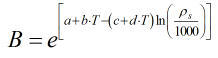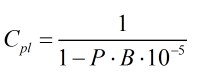# C223 – IP Paper 2 Compressibility and Cpl

## Description

This calculates the volume flow rate adjusted to take pressure and compressibility into account.

Kelton calculation reference C223

This calculation is used by the following calculations:

F029/K210 – IP Paper 2/Table 54 Density Referral

F022/K209 – IP Paper 2 Density Referral

Institute of Petroleum, Petroleum Measurement Paper No. 2; Guidelines for users of the petroleum measurement tables (API Std 2540; IP 200; ANSI/ASTM D 1250) (September 1984)

## Options

### Vapour Pressure

select this option to include the vapour pressure – the equilibrium bubble point pressure

This is a deviation from the standard which does not include vapour pressure. If this option is not selected is assumed that the vapour pressure is 1.01325 bar thus resulting in the ‘gauge’ value when subtracted from the absolute. This will then follow the process outlined in the standard.

### Rounding

The standard specifies the method of rounding/truncating , you can either select to round as per the standard or use the full precision.

a = 1.38315

b = 0.00343804

c = 3.02909

d = 0.0161654

## Calculations

### Step 1: Process the input variables

The pressure is truncated to three decimal places

The temperature is rounded to the nearest 0.05°C

The density is truncated to three decimal places

### Step 2: Initialise iteration parameters

Make initial estimate of density at 15°C

### Step 3: Calculate the compressibility factor from the equation### Step 4: Calculate the correction for pressure on liquid from the equationThis iterative calculation will be repeated with the recalculated value of standard density until convergence criteria are met.

Back